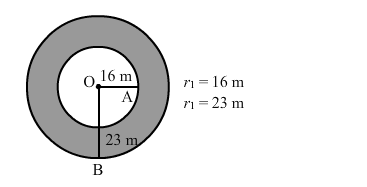# A rope by which a cow is tethered is increased from 16 m to 23 m.`
Question:

A rope by which a cow is tethered is increased from 16 m to 23 m. How much additional ground does it have now graze?

Solution:r1 = 16 m
r2 = 23 m

Amount of additional ground available $=$ Area of the bigger circle $-$ Area of the smaller circle

$=\pi\left(r_{1}^{2}-r_{2}^{2}\right)$

$=\pi\left(23^{2}-16^{2}\right)$

$=\pi(23+16)(23-16)$

$=858 \mathrm{~m}^{2}$